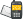It is currently 21 Sep 2020, 03:46### GMAT Club Daily Prep

#### Thank you for using the timer - this advanced tool can estimate your performance and suggest more practice questions. We have subscribed you to Daily Prep Questions via email.

Customized
for You

we will pick new questions that match your level based on your Timer History

Track

every week, we’ll send you an estimated GMAT score based on your performance

Practice
Pays

we will pick new questions that match your level based on your Timer History

#### Not interested in getting valuable practice questions and articles delivered to your email? No problem, unsubscribe here.# If d = 2c and e = a, what is x in terms of a, b, and c? (A)Question banks Downloads My Bookmarks Reviews Important topics
Author Message
TAGS:
Retired ModeratorJoined: 07 Jun 2014
Posts: 4803
GRE 1: Q167 V156WE: Business Development (Energy and Utilities)
Followers: 171

Kudos [?]: 2914 , given: 394

If d = 2c and e = a, what is x in terms of a, b, and c? (A) [#permalink]
Expert's post00:00

Question Stats:77% (02:01) correct22% (01:52) wrongbased on 18 sessions
Attachment:Capture.PNG [ 64.12 KiB | Viewed 1577 times ]

If $$d = 2c$$ and $$e = \frac{1}{2}a$$, what is x in terms of a, b, and c?

(A) $$\frac{3}{2}a + b + 3c - 540$$
(B) $$\frac{3}{2}a + b + 3c$$
(C) $$720 - \frac{3}{2}a - b - 3c$$
(D) $$720 - \frac{1}{2}a - b - 2c$$
(E) $$540 - \frac{1}{2}a - b - \frac{3}{2}c$$
[Reveal] Spoiler: OA

_________________

Sandy
If you found this post useful, please let me know by pressing the Kudos Button

Try our free Online GRE TestActive MemberJoined: 07 Jan 2018
Posts: 694
Followers: 11

Kudos [?]: 769  , given: 88

Re: If d = 2c and e = a, what is x in terms of a, b, and c? (A) [#permalink]
1
KUDOS
This is a six sided polygon. Among the six sides we know the five sides are given as variables. Let us suppose the sixth side is f. It follows f=180-x hence x=180-f

For six sided polygon total degrees inside the polygon is given by (n-2)180 = (6-2)180= 720

From this 720=a+b+c+d+e+f
or, 720-a-b-c-2c-1/2 a = f
replacing the value of d and e as mentioned in the question

Since x=180-f
x= 180-(720-a-b-c-2c-1/2 a)
rearranging the term we get x as option A

Posted from my mobile device_________________

This is my response to the question and may be incorrect. Feel free to rectify any mistakes
Need Practice? 20 Free GRE Quant Tests available for free with 20 KudosRe: If d = 2c and e = a, what is x in terms of a, b, and c? (A)   [#permalink] 20 Aug 2018, 05:40
Display posts from previous: Sort by

# If d = 2c and e = a, what is x in terms of a, b, and c? (A)Question banks Downloads My Bookmarks Reviews Important topicsPowered by phpBB © phpBB Group Kindly note that the GRE® test is a registered trademark of the Educational Testing Service®, and this site has neither been reviewed nor endorsed by ETS®.